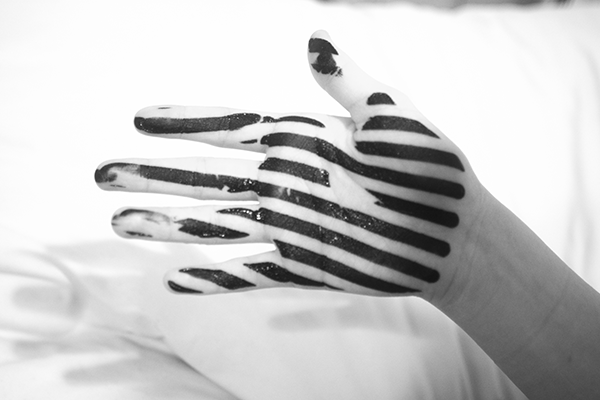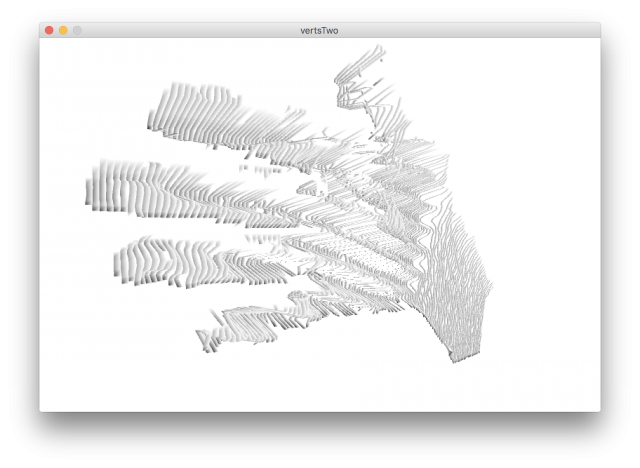# tyvan – Mocap

While learning about professional motion capture rigs during our lecture, I noted the high level techniques: reflective trackers are placed on the body for an IR camera setup to track in space. Software is able to find the high-value IR areas of the image, convert the micro areas to track points, align spatially similar dots, and turn that into a 3D model. I wanted to experiment with the first and final step of that process, converting value and light directly to 3D. The software used for this project is edited from the “MeshTweening” example in processing.Process:screen printed onto the model with shiny black ink, then photographed.2. The background of the images were removed by painting a 255 green over the areas.3. The Processing app was edited from the “MeshTweening” example to create
a desirable outcome. Placement on the Z-axis was defined by the value of the
represented pixel.

Although the code is interactive, and at some points animated, the final piece
is static imagery.

``` // Use of custom vertex attributes. // Inspired by // http://pyopengl.sourceforge.net/context/tutorials/shader_4.html   // Sets up Shader, Shape, and Values for Z-axis PShader sh; PShape grid[] = new PShape; PImage img; float imgValues[];   void setup() { size(800, 533, P3D);   // OpenGL Shader tools sh = loadShader("frag.glsl", "vert.glsl"); shader(sh);   // Image to render img = loadImage("________"); img.loadPixels();   int d = 5; // Line density unit for (int x = 0; x < img.width; x+=d) { int i = x/d; grid[i] = createShape();   // 'beginShape' function here allows Verticle lines, vs continuous surface grid[i].beginShape(); grid[i].noStroke(); for (int y = 0; y < img.height; y += d) {   //Convert to grayscale color colo = img.pixels[y * img.width + x]; fill(colo); float colorR = red(colo); float colorG = green(colo); float colorB = blue(colo); float value = (colorR + colorG + colorB)/3;   // Green Filter for input image if(colorG == 255){ grid[i].endShape(); grid[i].beginShape(); } else {   // Draw image in 3D Space as squares in columns grid[i].fill(255); grid[i].attribPosition("tweened", x, y, value); grid[i].vertex(x, y, 0);   grid[i].fill(255); grid[i].attribPosition("tweened", x + d, y, value); grid[i].vertex(x + d, y, 0);   grid[i].fill(255); grid[i].attribPosition("tweened", x + d, y + d, value ); grid[i].vertex(x + d, y + d, 0);   grid[i].fill(0); grid[i].attribPosition("tweened", x, y + d, value); grid[i].vertex(x, y + d, 0); } } grid[i].endShape(); } }   void draw() { background(255); int size = img.width/5;   // Allows for adjustment of perspective and composition pushMatrix(); translate(width/2, 0, 0); rotateX(mouseY*.005); rotateY(mouseX*.005); translate(0-width/2, 0, 0);   sh.set("tween", map(300, 0, width, 0, 1));   // Draw Shapes for (int i = 0; i < size; i++) { shape(grid[i]); } popMatrix(); }```